xIsentropic processEncyclopedia
In thermodynamics
Thermodynamics
Thermodynamics is a physical science that studies the effects on material bodies, and on radiation in regions of space, of transfer of heat and of work done on or by the bodies or radiation...

, an isentropic process or isoentropic process (ισον = "equal" (Greek); εντροπία entropy
Entropy
Entropy is a thermodynamic property that can be used to determine the energy available for useful work in a thermodynamic process, such as in energy conversion devices, engines, or machines. Such devices can only be driven by convertible energy, and have a theoretical maximum efficiency when...

= "disorder"(Greek)) is one in which for purposes of engineering analysis and calculation, one may assume that the process takes place from initiation to completion without an increase or decrease in the entropy of the system, i.e., the entropy of the system remains constant. It can be proven that any reversible
Reversible process (thermodynamics)
In thermodynamics, a reversible process, or reversible cycle if the process is cyclic, is a process that can be "reversed" by means of infinitesimal changes in some property of the system without loss or dissipation of energy. Due to these infinitesimal changes, the system is in thermodynamic...

adiabatic process
Adiabatic process
In thermodynamics, an adiabatic process or an isocaloric process is a thermodynamic process in which the net heat transfer to or from the working fluid is zero. Such a process can occur if the container of the system has thermally-insulated walls or the process happens in an extremely short time,...

is an isentropic process.

## Background

The Second law of thermodynamics
Second law of thermodynamics
The second law of thermodynamics is an expression of the tendency that over time, differences in temperature, pressure, and chemical potential equilibrate in an isolated physical system. From the state of thermodynamic equilibrium, the law deduced the principle of the increase of entropy and...

states that,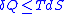where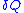is the amount of energy the system gains by heating,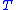is the temperature
Temperature
Temperature is a physical property of matter that quantitatively expresses the common notions of hot and cold. Objects of low temperature are cold, while various degrees of higher temperatures are referred to as warm or hot...

of the system, and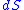is the change in entropy. The equal sign will hold for a reversible process
Reversible process (thermodynamics)
In thermodynamics, a reversible process, or reversible cycle if the process is cyclic, is a process that can be "reversed" by means of infinitesimal changes in some property of the system without loss or dissipation of energy. Due to these infinitesimal changes, the system is in thermodynamic...

. For a reversible isentropic process, there is no transfer of heat energy and therefore the process is also adiabatic
Adiabatic process
In thermodynamics, an adiabatic process or an isocaloric process is a thermodynamic process in which the net heat transfer to or from the working fluid is zero. Such a process can occur if the container of the system has thermally-insulated walls or the process happens in an extremely short time,...

. For an irreversible process, the entropy will increase. Hence removal of heat from the system (cooling) is necessary to maintain a constant internal entropy for an irreversible process in order to make it isentropic. Thus an irreversible isentropic process is not adiabatic.

For reversible processes, an isentropic transformation is carried out by thermally "insulating" the system from its surroundings. Temperature is the thermodynamic conjugate variable
Conjugate variables (thermodynamics)
In thermodynamics, the internal energy of a system is expressed in terms of pairs of conjugate variables such as temperature/entropy or pressure/volume. In fact all thermodynamic potentials are expressed in terms of conjugate pairs....

to entropy, thus the conjugate process would be an isothermal process
Isothermal process
An isothermal process is a change of a system, in which the temperature remains constant: ΔT = 0. This typically occurs when a system is in contact with an outside thermal reservoir , and the change occurs slowly enough to allow the system to continually adjust to the temperature of the reservoir...

in which the system is thermally "connected" to a constant-temperature heat bath.

## Isentropic flow

An isentropic flow is a flow
Fluid dynamics
In physics, fluid dynamics is a sub-discipline of fluid mechanics that deals with fluid flow—the natural science of fluids in motion. It has several subdisciplines itself, including aerodynamics and hydrodynamics...

that is both adiabatic and reversible. That is, no heat is added to the flow, and no energy transformations occur due to friction
Friction
Friction is the force resisting the relative motion of solid surfaces, fluid layers, and/or material elements sliding against each other. There are several types of friction:...

or dissipative effects
Dissipation
In physics, dissipation embodies the concept of a dynamical system where important mechanical models, such as waves or oscillations, lose energy over time, typically from friction or turbulence. The lost energy converts into heat, which raises the temperature of the system. Such systems are called...

. For an isentropic flow of a perfect gas, several relations can be derived to define the pressure, density and temperature along a streamline.

Note that energy can be exchanged with the flow in an isentropic transformation, as long as it doesn't happen as heat exchange. An example of such an exchange would be an isentropic expansion or compression, with entail work done on or by the flow.

### Derivation of the isentropic relations

For a closed system, the total change in energy of a system is the sum of the work done and the heat added,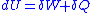The reversible work done on a system by changing the volume is,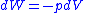where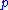is the pressure
Pressure
Pressure is the force per unit area applied in a direction perpendicular to the surface of an object. Gauge pressure is the pressure relative to the local atmospheric or ambient pressure.- Definition :...

and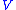is the volume. The change in enthalpy
Enthalpy
Enthalpy is a measure of the total energy of a thermodynamic system. It includes the internal energy, which is the energy required to create a system, and the amount of energy required to make room for it by displacing its environment and establishing its volume and pressure.Enthalpy is a...

(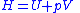) is given by,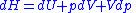Then for a process which is both reversible and adiabatic (i.e. no heat transfer occurs),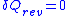, and so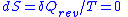. This leads to two important observations,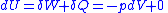, and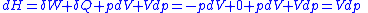Next, a great deal can be computed for isentropic processes of an ideal gas. For any transformation of an ideal gas, it is always true that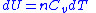, and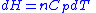.

Using the general results derived above for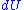and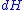, then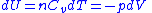, and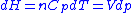.

So for an ideal gas, the heat capacity ratio
Heat capacity ratio
The heat capacity ratio or adiabatic index or ratio of specific heats, is the ratio of the heat capacity at constant pressure to heat capacity at constant volume . It is sometimes also known as the isentropic expansion factor and is denoted by \gamma or \kappa . The latter symbol kappa is...

can be written as,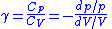For an ideal gas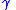is constant. Hence on integrating the above equation, assuming a perfect gas, we get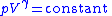i.e.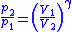Using the equation of state for an ideal gas,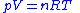,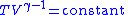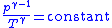also, for constant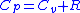(per mole),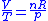and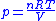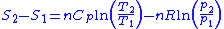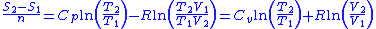Thus for isentropic processes with an ideal gas,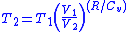or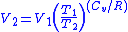### Table of isentropic relations for an ideal gas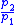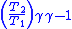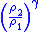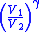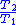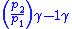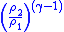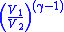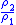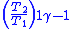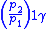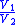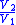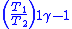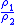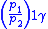Derived from: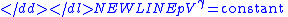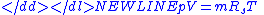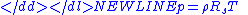Where: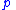= Pressure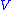= Volume= Ratio of specific heats =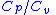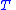= Temperature= Mass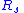= Gas constant for the specific gas =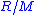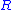= Universal gas constant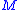= Molecular weight of the specific gas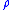= Density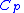= Specific heat at constant pressure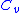= Specific heat at constant volume

## See also

• Adiabatic process
Adiabatic process
In thermodynamics, an adiabatic process or an isocaloric process is a thermodynamic process in which the net heat transfer to or from the working fluid is zero. Such a process can occur if the container of the system has thermally-insulated walls or the process happens in an extremely short time,...

• Isenthalpic process
Isenthalpic process
An isenthalpic process or isoenthalpic process is a process that proceeds without any change in enthalpy, H; or specific enthalpy, h....

• Polytropic process
The source of this article is wikipedia, the free encyclopedia.  The text of this article is licensed under the GFDL.